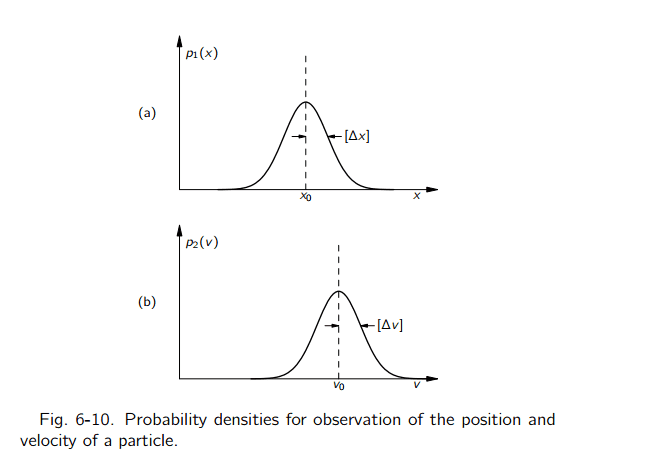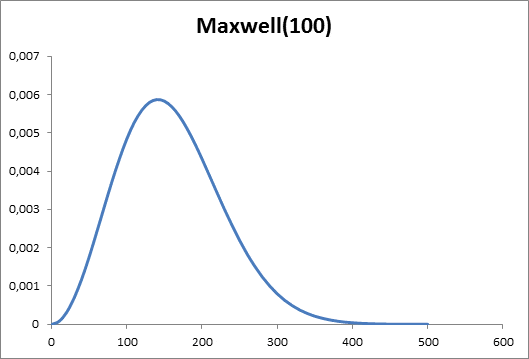# Probability density functions for velocity and position

• I
In the first volume of his lectures (cap. 6-5) Feynman asserts that these 2 can be the PDF of velocity and position of a particle.Under which conditions it's possible to model velocity and position of a particle using these particular PDFs ?

ps: Is the "Heisenberg uncertainty principle" applied only in the study of electrons and smaller particles ?

pps: Feynman also asserts that the $\Delta x$ and the $\Delta v$ in the "Heisenberg uncertainty principle" formula are two variances. Is this correct and accurate ?

#### Attachments

Last edited:

PeroK
Homework Helper
Gold Member
2021 Award
Under which conditions it's possible to model velocity and position of a particle using these particular PDFs ?

ps: Is the "Heisenberg uncertainty principle" applied only in the study of electrons and smaller particles ?

pps: Feynman also asserts that the $\Delta x$ and the $\Delta v$ in the "Heisenberg uncertainty principle" formula are two variances. Is this correct and accurate ?

The Heisenberg Uncertainty Principle is a statistical law that relates the variance of measurements of position and the variance of measurements of momentum of a particle in a given state.

If you know the state of a particle then that defines the position and momentum pdf's. But, those pdf's do not imply a definifite value for either position or momentum. There is always a non-zero variance for both. Then, the HUP says that:
$$\Delta x \Delta p \ge \frac{\hbar}{2}$$
Strictly speaking ##\Delta## here denotes the standard deviation, which is the square root of the variance.

The Heisenberg Uncertainty Principle is a statistical law that relates the variance of measurements of position and the variance of measurements of momentum of a particle in a given state.

If you know the state of a particle then that defines the position and momentum pdf's. But, those pdf's do not imply a definifite value for either position or momentum. There is always a non-zero variance for both. Then, the HUP says that:
$$\Delta x \Delta p \ge \frac{\hbar}{2}$$
Strictly speaking ##\Delta## here denotes the standard deviation, which is the square root of the variance.

But the PDF that describes the position and velocity of a particle are always normal ?

PeroK
Homework Helper
Gold Member
2021 Award
But the PDF that describes the position and velocity of a particle are always normal ?

Not always normal distributions, no. In theory the pdf of position can be anything. And, the pdf of momentum is the Fourier Transform of this.

Not always normal distributions, no. In theory the pdf of position can be anything. And, the pdf of momentum is the Fourier Transform of this.

Is it possible that Feynman use normal PDF since in the previous paragraph he introduced the Maxwell distribution of velocities of particles in a container, consequently he imagines to apply the HUP to the particles in the container ?

PeroK
Homework Helper
Gold Member
2021 Award
Is it possible that Feynman use normal PDF since in the previous paragraph he introduced the Maxwell distribution of velocities of particles in a container, consequently he imagines to apply the HUP to the particles in the container ?

I'm guessing that he intended nothing other than to illustrate the concept of a pdf and its standard deviation/variance.

I'm guessing that he intended nothing other than to illustrate the concept of a pdf and its standard deviation/variance.

But, if i observe the momentum of a particle based on the Maxwell Distribution of Velocity PDF, do i obtain a normal distribution ?

PeroK
Homework Helper
Gold Member
2021 Award
But, if i observe the momentum of a particle based on the Maxwell Distribution of Velocity PDF, do i obtain a normal distribution ?

I don't know.

I don't know.

What i think is that if i observe the momentum of a particle based on the Maxwell Distribution of Velocity PDF i don't obtain a normal distribution, but i get the Maxwell Distribution of Velocity PDF. So i still don't understand why Feynman use normal distributions of the observation of position and velocity of a particle...#### Attachments

What i think is that if i observe the momentum of a particle based on the Maxwell Distribution of Velocity PDF i don't obtain a normal distribution, but i get the Maxwell Distribution of Velocity PDF. So i still don't understand why Feynman use normal distributions of the observation of position and velocity of a particle...
As the mean velocity increases the Maxwell distribution gets ever closer to the normal distribution.
I think the point is that velocity and momentum become probability distributions linked by a FT. The Gaussian is a convenient pdf to use as the first example.

PeterDonis
Mentor
if i observe the momentum of a particle based on the Maxwell Distribution of Velocity PDF

What does this even mean? You don't observe "based on" any PDF. You just measure the momentum of a large number of identically prepared systems. Then, by looking at the set of measurement results, you find out what the PDF is for momentum for systems prepared that way.

PeterDonis
Mentor
i still don't understand why Feynman use normal distributions of the observation of position and velocity of a particle...

If one is a normal distribution, the other has to be, because the Fourier transform of a Gaussian is a Gaussian. So we can reduce this question to why Feynman picked a normal distribution for one of the two. The easier one to see is probably momentum, because in real experiments momentum of quantum systems is more often measured than position. And if you measure the momentum of a large number of identically prepared systems, unless the preparation process is unusual in some way, you are most likely to get a normal distribution of results simply by the law of large numbers. And Gaussians have a number of convenient mathematical properties. Those are probably the main reasons why Feynman used normal distributions.

And if you measure the momentum of a large number of identically prepared systems, unless the preparation process is unusual in some way, you are most likely to get a normal distribution of results simply by the law of large numbers.

So usually the momentum is measured as the average of many experiments in the same conditions ?

PeroK
Homework Helper
Gold Member
2021 Award
So usually the momentum is measured as the average of many experiments in the same conditions ?

The theory of QM is, generally, a statistical theory. The state of a system does not, in general, tell you what single value will result from a measurement, but the distribution of measurement values.

And If you ask whether there is a state that tells you precisely what you will measure for a set of observables, then in general there is no such state. The HUP quantifies this for position and momentum and the generalised UP quantifies it for any pair of observables.

Last edited:
•vanhees71